Computer Science

# Graph fundamentals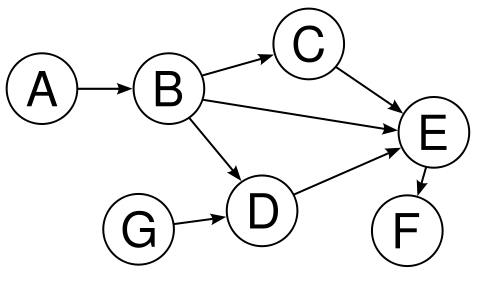For which of the following scenarios would a DAG (Directed acyclic graph) data structure be most appropriate?

Match the following graph with its corresponding adjacency matrix: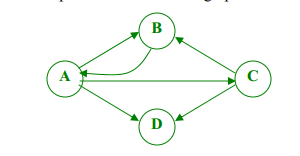A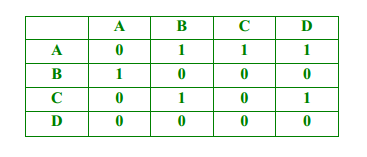B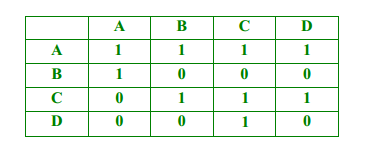C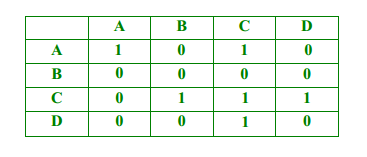D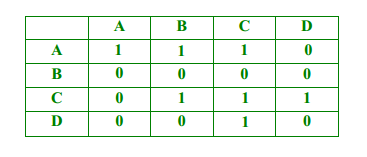Consider traversing the graph below using a depth-first search, beginning with vertex $1$. Which of the choices below lists vertices in the order they are visited in such a depth-first search traversal?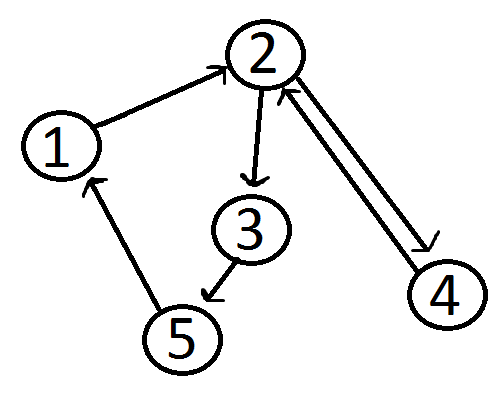Consider traversing the graph below using breadth-first search, beginning with vertex $1$. Which of the choices below lists vertices in the order they are visited in a breadth-first search traversal?Which of the following choices describes the graph below?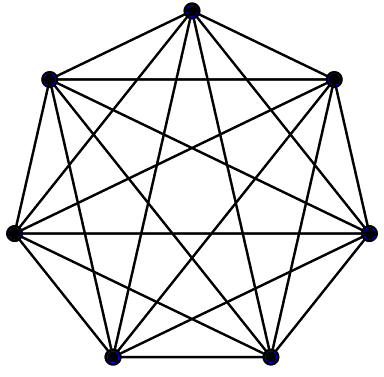×